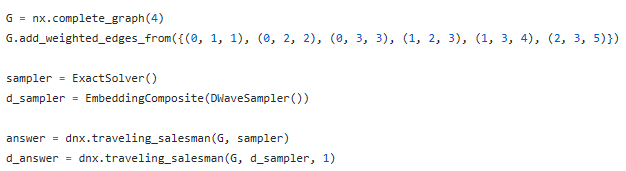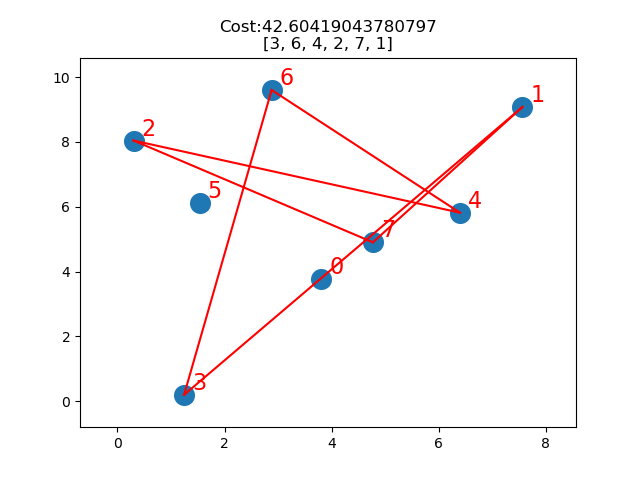# TSP (Traveling Salesman Problem)

Hey,

I'm working on a problem which can be transform to the TSP, so I want to using D-Wave  to solve it. But the API you provide(https://docs.ocean.dwavesys.com/projects/dwave-networkx/en/latest/reference/algorithms/generated/dwave_networkx.algorithms.tsp.traveling_salesman.html ) is only for small-scale TSP with the classical Solver not quantum Solver.

I want to know if a TSP with around 40 nodes can be solved by a Quantum Annealer. If could, can anyone provide some more detailed materials, such as websites, demos, or codes......

Andy

• Hi Andy,

This is totally possible!
To use the QPU, you just need to use a different solver:
from dwave.system.samplers import DWaveSampler
solver=DWaveSampler(solver=my_solver, token=my_dwave_token)

For larger problems you just need to add more nodes like in the example.

Please let us know if this helped!

• Hi Andy,

Michal Stechly has written a TSP solver using the D-Wave QPU. He has just put up an article describing it a few weeks ago. The article includes a link to his code and a demo.

Ed

• Hi, I am very grateful for your answers. I have tested the example using DWaveSampler(), but I got unpredictable results - sometimes long list - sometimes list of length one, comparing with the ExactSolver(). I don’t why. Codes reference Fig.1.

I have run the TSP demo written by Michal Stechly, but the nodes of TSP more than about 10 could not get the result and return a “ValueError: no embedding found” error in Python. After many times tests for this demo using D-Wave, the results of TSP with 5 to 10 nodes were apparently wrong.(Fig.2)

So if you have any great solutions about these problems, please tell me how you solved it.

Fig.1:Fig.2:• Hi Andy,

At present the traveling salesperson problem for 10 cities converts into a model that has over 100 variables.

Variables correspond to paths between cities.

This is a very big problem that can not be embedded directly onto the QPU.

At present the QPU can embed a maximum of 8 cities in this way.

The code is open source, so it is possible to look at the implementation and do some clever rearranging to change how the program is represented on the QPU:
https://docs.ocean.dwavesys.com/projects/dwave-networkx/en/latest/_modules/dwave_networkx/algorithms/tsp.html

This will be a bit difficult and require a bit more understanding of how the QPU works.

Here are some links to the tutorial by Michal Stechly that another user mentioned above:

Here is the main page:
https://github.com/mstechly/quantum_tsp_tutorials

This looks like the intro to the Traveling Salesperson tutorial:
https://github.com/mstechly/quantum_tsp_tutorials/blob/master/tutorials/01_Introduction_to_TSP.ipynb

• Andy,
I am still learning about how to use D-Wave's qpu on larger problems, but this is what I think is happening. You are encountering two practical issues which occur when working with the QPU as the problem size grows: 1) The probabilistic
nature of the QPU and 2) the models of many problems are larger than what can be embedded in the D-Wave 2000Q architecture directly.

1) The QPU solver is noisy and probabilistic in nature and returns a sample of potential solutions, not necessarily valid solutions.

You will want to iterate through the list of returned solutions and choose only valid ones. In the TSP example, if you you look at the code on https://docs.ocean.dwavesys.com/projects/dwave-networkx/en/latest/_modules/dwave_networkx/algorithms/tsp.html   , you will see there an is_hamiltonian_path function which can be used to verify each solution returned by the sampler (or your own validation function) and filter your results accordingly.

2) Many problems are larger than what can be embedded directly in the D-Wave 2000Q architecture.

As you have found, the TSP model you are working with cannot handle more than 10 cities. To handle larger problems, we need to find a way to decompose the problem into smaller sub-problems which can be handled and as a final step, compose a final solution from the solutions to each of the sub-problems.

I haven't done this yet, but the next step I would try would be to use the dwave-hybrid decomposers and composers, similar to what is used in https://github.com/dwavesystems/dwave-hybrid/blob/master/examples/qbsolv-like.py .

As I mentioned above, I am still learning, so if anyone sees I have written something incorrect here, please let me know. I have thick skin.

Ed

• I highly recommend the paper:  Sebastin Feld et al., “A Hybrid Solution Method for the Capacitated Vehicle Routing Problem Using a Quantum Annealer”, (2018), arXiv:1811.07403v1.  In Table 1 the authors report quantum annealing results for solving TSPs with 14, 16, 22, 29 and 38 cities.  The quantum results for 14 and 16 cities are the exact distances for an optimal tour.  The best quantum results for the other three TSPs are approximate.  The report indicates 100 executions per TSP and 250 samples per execution.

Richard W.